7 days of WordPress plugins, themes & templates - for free!* Unlimited asset downloads! Start 7-Day Free Trial

# Quick Tip: Collision Detection Between a Circle and a Line SegmentRead Time: 6 mins

We covered collision detection between an infinite line and circle in our previous Quick Tip. However, the issue that arose was that the line extends further than the visible line segment; in fact, it extends into a hyperplane. In this Quick Tip, we shall limit our collision detection to that of a line segment only.

## Final Result Preview

We shall work toward this result:

Click the Restart button to reposition the circles at the top of the stage.

## Step 1: Two Approaches

There are numerous approaches to limit collision detection to within a line segment. We shall look at two approaches this time. The first approach is a little more rigorous mathematically than the second one, but these are concepts which, if you grasp successfully, will surely benefit you in the future. Both approaches manipulate the dot product's characteristic of being a measure of how parallel two given vectors are.

Let's have a look at the first approach. Suppose A and B are vectors. If A and B are parallel - or at least pointing in the same direction - the dot product between A and B will produce a positive number. If A and B are pointing directly opposite each other - or at least pointing in opposing directions - the dot product between A and B will produce a negative number. If A and B are orthogonal (forming 90° to each other) then the dot product will produce 0.

The diagram below summarises this description.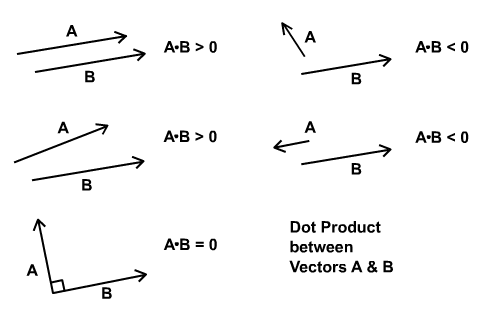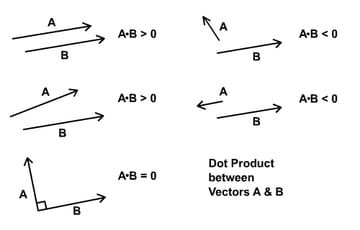## Step 2: Relate Dot Product to Conditions

We'll need to form vectors B and C from both ends of the line segment so that their dot product with the line segment's vector, A, can determine whether the circle is within the segment.

Observe the diagram below. If the circle is within the segment, then the value of the dot product between A and B is positive and that between A and C is negative.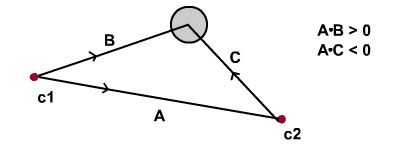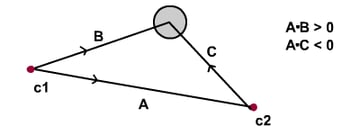The diagram below shows how the dot product changes depending on whether the circle is beyond or within the line segment. Note the differences in the value of the dot product.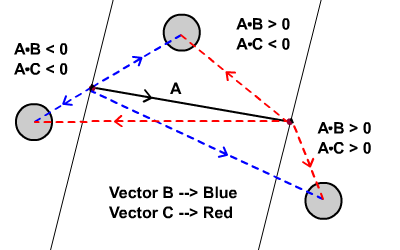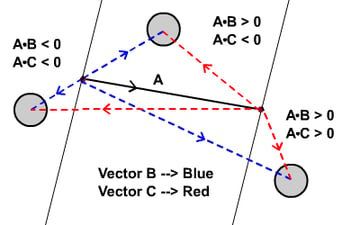Also note that "within the line segment" does not mean that the circle is necessarily intersecting the line segment, just that it falls within the two thin lines on the diagram above.

So when collision occurs between line and circle, as we have seen in the previous Quick Tip, we have to further investigate whether the circle is positioned within the line segment. If it is, then we know for sure that there is a genuine intersection.

## Step 3: Implementation

Step 2 explained the concept we use to restrict collision detection to be within the line segment. However, there's still a flaw in the precision. You see, the area defined is a little tilted; we should aim to use the area defined according to the diagram below.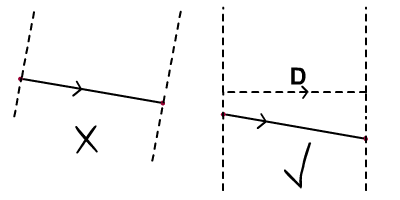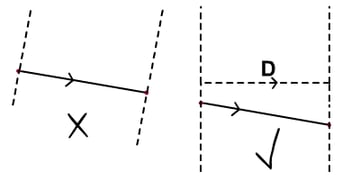This is easy: we simply calculate D as the horizontal projection of A. Then, instead of using A, we use D to dot product with B and C. All the conditions as explained in Step 2 still stand, but instead of a tilted segment, we have defined a vertical area.

This correction can be visually appreciated if the circle is large; if the circle were small, its center would be so close to the line that this visual flaw would be hard to detect, so we could get away with using that slightly tilted area and save ourselves some processing power.

Nevertheless, I'll try to do things the correct way. You can pick your approach by modifying the condition slightly.

## Step 4: Implementation

The first Actionscript snippet here sets up vector D (v_line_onX)

Note: We're using classes from my previous tutorials here. Vector2D was introduced in Gravity in Action, but you don't need to read that to use the class, it's included in the source download.

The second Actionscript snippet here sets up B (c1_circle) and C (c2_circle) and checks for the collision and whether the circle is inside the segment or not.

## Step 5: The Result

Here's the result for the first approach. Click on the button to reset positions of all circles to the top of stage.

## Step 6: Second Approach

The second approach is much simpler. I'll try to work backwards from the end this time around.

Observe the diagram below. The line segment is from c1 to c2. It's clear that collide1 and collide3 are both outside the line segment, and that only collide2 is within the line segment.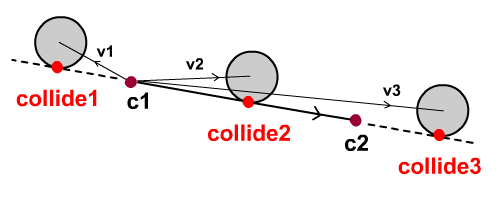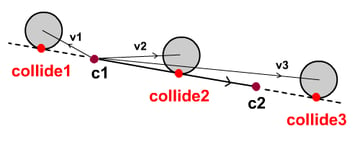Let v1, v2 and v3 be vectors from c1 to respective circles. Only v2 and v3 are parallel - or at least pointing in similar directions to the line vector (c1 to c2). By checking for a positive value in the dot product between the line vector and each of those vectors from c1 to the corresponding circle centers (v1, v2, v3), we can easily determine that collide1 is beyond the line segment. In other words, c1 . v1 < 0.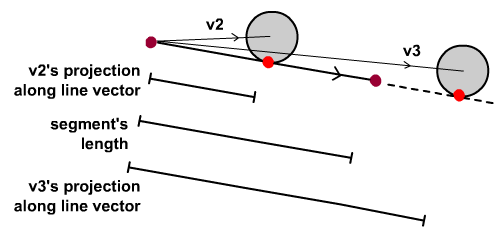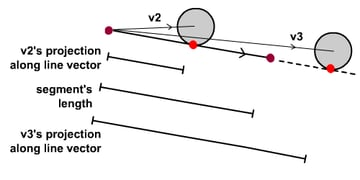Next, we shall devise a method to determine that collide3 is outside of the line segment. This should be easy. It's obvious that v3's projection along the line vector will exceed the length of line segment. We shall use this characteristic to weed off collide3.

So let me summarise the second approach:

• First we check for an intersection between the infinite line and the circle.
• If there is an intersection, further investigate the following to determine whether it happens within the line segment:
• Check that a positive value is produced when we take the dot product of the vector from c1 to circle and the line vector, and
• Check that the magnitude of the projection of the vector along the line vector is shorter than the line segment's length.

## Step 7: Implementation

Here's the ActionScript implementation of the above:

## Step 8: The Result

Essentially, it will produce the same result as the previous but since there is a few lines of code shorter in the second approach, I guess its better.

## Conclusion

Hope this has helped. Thanks for reading. Next up, we'll look at collision reaction.